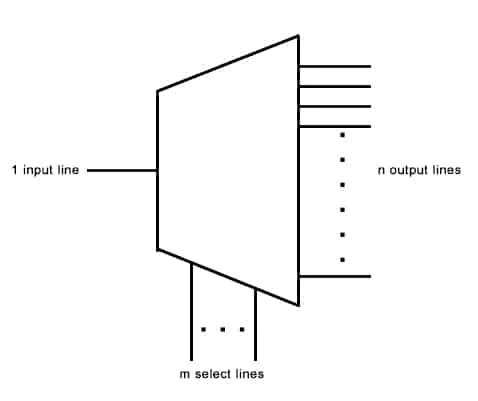View Course Path

# VHDL code for demultiplexer using dataflow method – full code & explanation

Next up in this VHDL course, we are going to write the VHDL code for demultiplexer using the dataflow architecture. We will model the 1×2 demux using logic equations, write its testbench, generate simulation waveforms and RTL schematic. And then we will do the same for a 1×4 mux, albeit with one difference. We will use the truth table instead of logic equations for the VHDL code. Let’s revisit the demultiplexer briefly before we begin.

Demultiplexer

A demultiplexer is a combinational digital logic circuit that assigns one input to one of several output lines. It selects one of these output lines depending on the value of its select inputs. So a demultiplexer has one input signal, select lines, and multiple output lines.This is a general Demultiplexer with its single input, multiple outputs, and corresponding select lines.

Contents

## Using Logic Equations

#### Logic equation for a 1:2 Demux

Y0 = I0S’

Y1 = I0S

Here, I0 is the input. S is the select line input. Y0 and Y1 are the outputs. To see how we got this equation, you can check out the working of the 1:2 demux.

### VHDL code for demultiplexer using dataflow (logic equation) method – 1:2 Demux

```library IEEE;
use IEEE.STD_LOGIC_1164.ALL;
use IEEE.STD_LOGIC_ARITH.ALL;
use IEEE.STD_LOGIC_UNSIGNED.ALL;

entity DEMUX_SOURCE is
Port ( I,S : in  STD_LOGIC;
O1, O2 : out  STD_LOGIC);
end DEMUX_SOURCE;

architecture dataflow of DEMUX_SOURCE is

begin

O1 <= I and (not S);
O2 <= I and S;

end dataflow;```

### Testbench

```library IEEE;
use IEEE.STD_LOGIC_1164.ALL;
use IEEE.STD_LOGIC_ARITH.ALL;
use IEEE.STD_LOGIC_UNSIGNED.ALL;

entity demux_tb is
end entity;

architecture tb of demux_tb is
component DEMUX_SOURCE is
Port ( I,S : in STD_LOGIC;
O1, O2 : out STD_LOGIC);
end component;

signal I, S, O1, O2 : STD_LOGIC;

begin

uut: DEMUX_SOURCE port map(
I => I, S => S,
O1 => O1, O2 => O2);

stim: process
begin

I <= '0';

S <= '0';
wait for 20 ns;
S <= '1';
wait for 20 ns;

I <= '1';

S <= '0';
wait for 20 ns;
S <= '1';
wait for 20 ns;

wait;

end process;
end tb;```

In case you’d like to brush up a few concepts, here is our guide on writing a testbench using VHDL.

### RTL Schematic### Simulation Waveforms## Using Truth Table

#### Truth table of 1:4 demux

 I0 S0 S1 Y0 Y1 Y2 Y3 I 0 0 I 0 0 0 I 0 1 0 I 0 0 I 1 0 0 0 I 0 I 1 1 0 0 0 I

## VHDL code for demultiplexer using dataflow (truth table) method – 1:4 Demux

Usually, we see the truth table is used to code in the behavioral architecture. However, it is possible to use the truth table of a digital electronic circuit in the dataflow architecture too. This demux code is a perfect example of doing that. We’ll be using the truth table to write the demultiplexer’s VHDL source code. We will proceed just like we did with the with-select statements in the VHDL coding for multiplexers.

```library IEEE;
use IEEE.STD_LOGIC_1164.ALL;
use IEEE.STD_LOGIC_ARITH.ALL;
use IEEE.STD_LOGIC_UNSIGNED.ALL;

entity DEMUX_USINGTRUTHTABLE_SOURCE is
Port ( I : in  STD_LOGIC;
S : in  STD_LOGIC_VECTOR (1 downto 0);
Y : out  STD_LOGIC_VECTOR (3 downto 0));
end DEMUX_USINGTRUTHTABLE_SOURCE;

architecture dataflow of DEMUX_USINGTRUTHTABLE_SOURCE is

begin
--- since the output is a combination of character and binary bits, bitwise operation with bit and CHARACTER will take place (&) not logical and
with S select

Y              <=               ("000" & I) when "00",
("00" & I & "0") when "01",
("0" & I & "00") when "10",
(I & "000") when others;

end dataflow;```

### Testbench

```library IEEE;
use IEEE.STD_LOGIC_1164.ALL;
use IEEE.STD_LOGIC_ARITH.ALL;
use IEEE.STD_LOGIC_UNSIGNED.ALL;

entity Demultiplexer_tb is
end entity;

architecture tb of Demultiplexer_tb is
component DEMUX_USINGTRUTHTABLE_SOURCE is
Port ( I : in STD_LOGIC;
S : in STD_LOGIC_VECTOR (1 downto 0);
Y : out STD_LOGIC_VECTOR (3 downto 0));
end component;

signal I : STD_LOGIC;
signal S : STD_LOGIC_VECTOR(1 downto 0);
signal Y : STD_LOGIC_VECTOR(3 downto 0);

begin

uut: DEMUX_USINGTRUTHTABLE_SOURCE port map(
I => I,
S => S,
Y => Y);

stim: process
begin

I <= '1';
S <= "00";
wait for 20 ns;

S <= "01";
wait for 20 ns;

S <= "10";
wait for 20 ns;
S <= "11";
wait for 20 ns;

wait;

end process;
end tb;```

## RTL Schematic## Simulation WaveformsWere you able to write the VHDL code for your demux? Let us know what you did differently by commenting below. Also, would you like us to include the VHDL code for a 1:8 demux?

Edit: Post updated with the testbench, RTL Schematic, and Simulation Waveform by Deepak Joshi.

This site uses Akismet to reduce spam. Learn how your comment data is processed.

Top